1. /
2. CBSE
3. /
4. Class 06
5. /
6. Mathematics
7. /
8. NCERT Solutions for Class...

# NCERT Solutions for Class 6 Maths Exercise 14.6

NCERT solutions for Maths Practical geometry## NCERT Solutions for Class 6 Maths Practical geometry

###### Question 1.Draw {tex}\angle {/tex}POQ of measure {tex}75^\circ {/tex} and find its line of symmetry.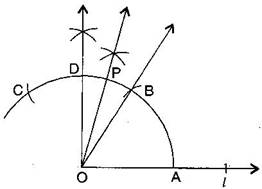(a) Draw a line {tex}l{/tex} and mark a point O on it.

(b) Place the pointer of the compasses at O and draw an arc of any radius which intersects the line {tex}l{/tex} at A.

(c) Taking same radius, with centre A, cut the previous arc at B.

(d) Join OB, then {tex}\angle {/tex}BOA = {tex}{60^ \circ }.{/tex}

(e) Taking same radius, with centre B, cut the previous arc at C.

(f) Draw bisector of {tex}\angle {/tex}BOC. The angle is of {tex}{90^ \circ }.{/tex} Mark it at D. Thus, {tex}\angle {/tex}DOA = {tex}{90^ \circ }{/tex}

(g) Draw {tex}\overline {{\text{OP}}} {/tex} as bisector of {tex}\angle {/tex}DOB.

Thus, {tex}\angle {/tex}POA = {tex}75^\circ {/tex}

###### Question 2.Draw an angle of measure {tex}147^\circ {/tex} and construct its bisector.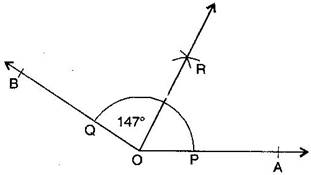(a) Draw a ray {tex}\overleftrightarrow {{\text{OA}}}.{/tex}

(b) With the help of protractor, construct {tex}\angle {/tex}AOB = {tex}147^\circ .{/tex}

(c) Taking centre O and any convenient radius, draw an arc which intersects the arms {tex}\overline {{\text{OA}}} {/tex} and {tex}\overline {{\text{OB}}} {/tex} at P and Q respectively.

(d) Taking P as centre and radius more than half of PQ, draw an arc.

(e) Taking Q as centre and with the same radius, draw another arc which intersects the previous at R.

(f) Join OR and produce it.

Thus, {tex}\overline {{\text{OR}}} {/tex} is the required bisector of {tex}\angle {/tex}AOB.

NCERT Solutions for Class 6 Maths Exercise 14.6

###### Question 3.Draw a right angle and construct its bisector.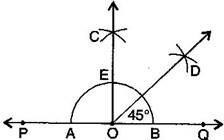(a) Draw a line PQ and take a point O on it.

(b) Taking O as centre and convenient radius, draw an arc which intersects PQ at A and B.

(c) Taking A and B as centers and radius more than half of AB, draw two arcs which intersect each other at C.

(d) Join OC. Thus, {tex}\angle {/tex}COQ is the required right angle.

(e) Taking B and E as centre and radius more than half of BE, draw two arcs which intersect each other at the point D.

(f) Join OD. Thus, {tex}\overline {{\text{OD}}} {/tex} is the required bisector of {tex}\angle {/tex}COQ.

NCERT Solutions for Class 6 Maths Exercise 14.6

###### Question 4. Draw an angle of measure {tex}153^\circ {/tex} and divide it into four equal parts.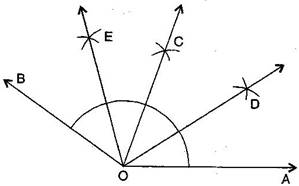(a) Draw a ray {tex}\overleftrightarrow {{\text{OA}}}.{/tex}

(b) At O, with the help of a protractor, construct {tex}\angle {/tex}AOB = {tex}153^\circ .{/tex}

(c) Draw {tex}\overline {{\text{OC}}} {/tex} as the bisector of {tex}\angle {/tex}AOB.

(d) Again, draw {tex}\overline {{\text{OD}}} {/tex} as bisector of {tex}\angle {/tex}AOC.

(e) Again, draw {tex}\overline {{\text{OE}}} {/tex} as bisector of {tex}\angle {/tex}BOC.

(f) Thus, {tex}\overline {{\text{OC}}} {/tex}, {tex}\overline {{\text{OD}}} {/tex} and {tex}\overline {{\text{OE}}} {/tex} divide {tex}\angle {/tex}AOB in four equal arts.

NCERT Solutions for Class 6 Maths Exercise 14.6

###### Question 5.Construct with ruler and compasses, angles of following measures:

(a) {tex}{60^ \circ }{/tex}

(b) {tex}{30^ \circ }{/tex}

(c) {tex}{90^ \circ }{/tex}

(d) {tex}{120^ \circ }{/tex}

(e) {tex}{45^ \circ }{/tex}

(f) {tex}{135^ \circ }{/tex}

Steps of construction: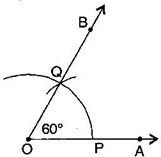(a) {tex}{60^ \circ }{/tex}

(i) Draw a ray {tex}\overleftrightarrow {{\text{OA}}}.{/tex}

(ii) Taking O as centre and convenient radius, mark an arc, which intersects {tex}\overleftrightarrow {{\text{OA}}}{/tex} at P.

(iii) Taking P as centre and same radius, cut previous arc at Q.

(iv) Join OQ.

Thus, {tex}\angle {/tex}BOA is required angle of {tex}{60^ \circ }.{/tex}

(b) {tex}{30^ \circ }{/tex}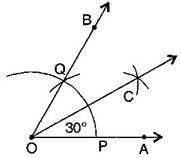(i) Draw a ray {tex}\overleftrightarrow {{\text{OA}}}.{/tex}

(ii) Taking O as centre and convenient radius, mark an arc, which intersects {tex}\overleftrightarrow {{\text{OA}}}{/tex} at P.

(iii) Taking P as centre and same radius, cut previous arc at Q.

(iv) Join OQ. Thus, {tex}\angle {/tex}BOA is required angle of {tex}{60^ \circ }.{/tex}

(v) Put the pointer on P and mark an arc.

(vi) Put the pointer on Q and with same radius, cut the previous arc at C.

Thus, {tex}\angle {/tex}COA is required angle of {tex}{30^ \circ }.{/tex}

(c){tex}{90^ \circ }{/tex}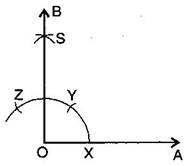(i) Draw a ray {tex}\overleftrightarrow {{\text{OA}}}.{/tex}

(ii) Taking O as centre and convenient radius, mark an arc, which intersects {tex}\overleftrightarrow {{\text{OA}}}{/tex} at X.

(iii) Taking X as centre and same radius, cut previous arc at Y.

(iv) Taking Y as centre and same radius, draw another arc intersecting the same arc at Z.

(v) Taking Y and Z as centers and same radius, draw two arcs intersecting each other at S.

(vi) Join OS and produce it to form a ray OB.

Thus, {tex}\angle {/tex}BOA is required angle of {tex}{90^ \circ }.{/tex}

(d){tex}{120^ \circ }{/tex}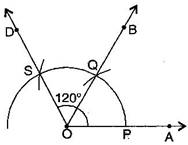(i) Draw a ray {tex}\overleftrightarrow {{\text{OA}}}.{/tex}

(ii) Taking O as centre and convenient radius, mark an arc, which intersects {tex}\overleftrightarrow {{\text{OA}}}{/tex} at P.

(iii) Taking P as centre and same radius, cut previous arc at Q.

(iv) Taking Q as centre and same radius cut the arc at S.

(v) Join OS.

Thus, {tex}\angle {/tex}AOD is required angle of {tex}{120^ \circ }.{/tex}

(e){tex}{45^o}{/tex}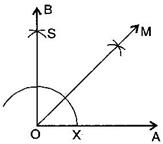(i) Draw a ray {tex}\overrightarrow {OA} {/tex}

(ii) Taking O as centre and convenient radius, mark an arc, which intersects {tex}\overrightarrow {OA} {/tex} at X.

(iii) Taking X as centre and same radius, cut previous arc at Y.

(iv) Taking Y as centre and same radius, draw another arc intersecting the same arc at Z.

(v) Taking Y and Z as centers and same radius, draw two arcs intersecting each other at S.

(vi) Join OS and produce it to form a ray OB. Thus, {tex}\angle {/tex}BOA is required angle of {tex}{90^o}{/tex}

(vii) Draw the bisector of {tex}\angle {/tex}BOA.

Thus, {tex}\angle {/tex}MOA is required angle of {tex}{45^o}{/tex}

(f){tex}{135^o}{/tex}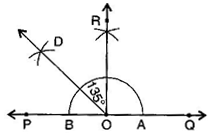(i) Draw a line PQ and take a point O on it.

(ii) Taking O as centre and convenient radius, mark an arc, which intersects PQ at A and B.

(iii) Taking A and B as centers and radius more than half of AB, draw two arcs intersecting each other at R.

(iv) Join OR. Thus, {tex}\angle {/tex}QOR = {tex}\angle {/tex}POQ = {tex}{90^o}{/tex}

(v) Draw {tex}\overrightarrow {OD} {/tex} the bisector of {tex}\angle {/tex}POR.

thus, {tex}\angle {/tex}QOD is required angle of {tex}{135^o}{/tex}

NCERT Solutions for Class 6 Maths Exercise 14.6

###### Question 6.Draw an angle of measure {tex}{45^ \circ }{/tex} and bisect it.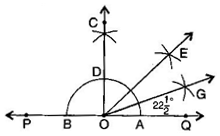(a)Draw a line PQ and take a point O on it.

(b)Taking O as centre and a convenient radius, draw an arc which intersects PQ at two points A and B.

(c)Taking A and B as centers and radius more than half of AB, draw two arcs which intersect each other at C.

(d)Join OC. Then {tex}\angle {/tex}COQ is an angle of {tex}{90^o}{/tex}

(e)Draw {tex}\overrightarrow {OE} {/tex} as the bisector of {tex}\angle {/tex}COE. Thus, {tex}\angle {/tex}QOE = {tex}{45^o}{/tex}

(f)Again draw {tex}\overrightarrow {OG} {/tex} as the bisector of {tex}\angle {/tex}QOE.

Thus, {tex}\angle {/tex}QOG = {tex}\angle {/tex}EOG = {tex}22\frac{1}{2}{\,^o}{/tex}

NCERT Solutions for Class 6 Maths Exercise 14.6

###### Question 7.Draw an angle of measure {tex}{135^ \circ }{/tex} and bisect it.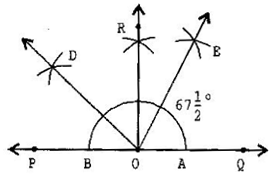(a) Draw a line PQ and take a point O on it.

(b) Taking O as centre and convenient radius, mark an arc, which intersects PQ at A and B.

(c) Taking A and B as centers and radius more than half of AB, draw two arcs intersecting each other at R.

(d) Join OR. Thus, {tex}\angle {/tex}QOR = {tex}\angle {/tex}POQ = {tex}{90^o}{/tex}

(e) Draw {tex}\overrightarrow {OD} {/tex} the bisector of {tex}\angle {/tex}POR. Thus, {tex}\angle {/tex}QOD is required angle of {tex}{135^o}{/tex}

(f) Now, draw {tex}\overrightarrow {OE} {/tex} as the bisector of {tex}\angle {/tex}QOD.

Thus, {tex}\angle {/tex}QOE = {tex}\angle {/tex}DOE = {tex}67\frac{1}{2}{\,^o}{/tex}

NCERT Solutions for Class 6 Maths Exercise 14.6

###### Question 8.Draw an angle of {tex}70^\circ .{/tex} Make a copy of it using only a straight edge and compasses.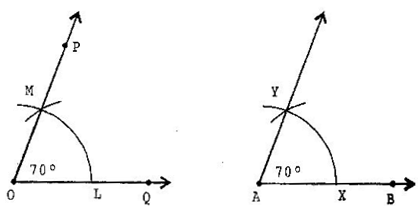(a) Draw an angle {tex}{70^o}{/tex} with protractor, i.e., {tex}\angle POQ = {70^o}{/tex}

(b) Draw a ray {tex}\overrightarrow {AB} {/tex}

(c) Place the compasses at O and draw an arc to cut the rays of {tex}\angle {/tex}POQ at L and M.

(d) Use the same compasses, setting to draw an arc with A as centre, cutting AB at X.

(e) Set your compasses setting to the length LM with the same radius.

(f) Place the compasses pointer at X and draw the arc to cut the arc drawn earlier at Y.

(g) Join AY.

Thus, {tex}\angle YAX = {70^o}{/tex}

NCERT Solutions for Class 6 Maths Exercise 14.6

###### Question 9.Draw an angle of {tex}40^\circ .{/tex} Copy its supplementary angle.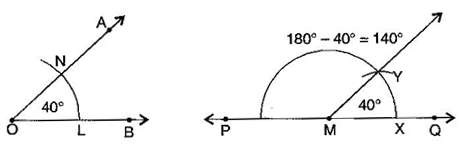(a) Draw an angle of {tex}{40^o}{/tex} with the help of protractor, naming {tex}\angle {/tex}AOB.

(b) Draw a line PQ.

(c) Take any point M on PQ.

(d) Place the compasses at O and draw an arc to cut the rays of {tex}\angle {/tex}AOB at L and N.

(e) Use the same compasses setting to draw an arc O as centre, cutting MQ at X.

(g) Place the compasses at X and draw the arc to cut the arc drawn earlier Y.

(h) Join MY.

Thus, {tex}\angle {/tex}QMY = {tex}{40^o}{/tex} and {tex}\angle {/tex}PMY is supplementary of it.

## NCERT Solutions for Class 6 Maths Exercise 14.6

NCERT Solutions Class 6 Maths PDF (Download) Free from myCBSEguide app and myCBSEguide website. Ncert solution class 6 Maths includes text book solutions from Class 6 Maths Book . NCERT Solutions for CBSE Class 6 Maths have total 14 chapters. 6 Maths NCERT Solutions in PDF for free Download on our website. Ncert Maths class 6 solutions PDF and Maths ncert class 6 PDF solutions with latest modifications and as per the latest CBSE syllabus are only available in myCBSEguide.

## CBSE app for Students

To download NCERT Solutions for Class 6 Maths, Social Science Computer Science, Home Science, Hindi English, Maths Science do check myCBSEguide app or website. myCBSEguide provides sample papers with solution, test papers for chapter-wise practice, NCERT solutions, NCERT Exemplar solutions, quick revision notes for ready reference, CBSE guess papers and CBSE important question papers. Sample Paper all are made available through the best app for CBSE students and myCBSEguide website.### Test Generator

Create question paper PDF and online tests with your own name & logo in minutes.### myCBSEguide

Question Bank, Mock Tests, Exam Papers, NCERT Solutions, Sample Papers, Notes

### 4 thoughts on “NCERT Solutions for Class 6 Maths Exercise 14.6”

1. So good site ???

2. i hate you

3. Good

4. So cool man